## How to Construct an Equilateral Triangle?: Geometry

Happy Wednesday math peeps! This post introduces constructions by showing us how to construct an equilateral triangle by using a compass and a ruler. For anyone new to constructions, this is the perfect topic for art aficionados since there is more drawing than there is actual math.

## What is an Equilateral Triangle?

Equilateral Triangle: A triangle with three equal sides.  Not an easy one to forget, the equilateral triangle is super easy to construct given the right tools (compass+ ruler). Take a look below:

Now, for our Example:

## What’s Happening in this GIF?

1. Using a compass, measure out the distance of line segment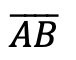.

2. With the compass on point A, draw an arc that has the same distance as.

3. With the compass on point B, draw an arc that has the same distance as.

4. Notice where the arcs intersect? Using a ruler, connect points A and B to the new point of intersection. This will create two new equal sides of our triangle!

Still got questions? No problem! Don’t hesitate to comment with any questions. Happy calculating! 🙂

## Reflections: Geometry

Greetings and welcome to Mathsux! Today we are going to go over reflections, one of the many types of transformations that come up in geometry.  And thankfully, it is one of the easiest transformation types to master, especially if you’re more of a visual learner/artistic type person. So let’s get to it!

## What are Reflections?

Reflections on a coordinate plane are exactly what you think! When a point, a line segment, or a shape is reflected over a line it creates a mirror image.  Think the wings of a butterfly, a page being folded in half, or anywhere else where there is perfect symmetry.

## Example:

Step 1: First, let’s draw in line x=-2.

Step 2: Find the distance each point is from the line x=-2 and reflect it on the other side, measuring the same distance. First, let’s look at point C, notice it’s 1 unit away from the line x=-2, to reflect it we are going to count 1 unit to the left of the line x=-2 and label our new point, C|.

Step 3: Next we reflect point A in much the same way! Notice that point A is 2 units away on the left of line x=-2, we then measure 2 units to the right of our line and mark our new point, A|.

Step 4: Lastly, we reflect point B. This time, point B is 1 unit away on the right side of the line x=-2, we then measure 1 unit to the opposite side of our line and mark our new point, B|.

Step 5: Finally, we can now connect all of our new points, for our fully reflected triangle A|B|C|.

## Solutions:

Still got questions?  No problem! Check out the video above or comment below! Happy calculating! 🙂

Looking to review rotations about a point? Check out this post here!

## Piecewise Functions: Algebra

Greetings, today’s post is for those in need of a piecewise functions review!  This will cover how to graph each part of that oh so intimidating piecewise functions.  There’s x’s, there are commas, there are inequalities, oh my! We’ll figure out what’s going on here and graph each part of the piecewise-function one step at a time.  Then check yourself with the practice questions at the end of this post. Happy calculating! 🙂

## What are Piece-Wise Functions?

Exactly what they sound like! A function that has multiple pieces or parts of a function.  Notice our function below has different pieces/parts to it.  There are different lines within, each with their own domain.

Now let’s look again at how to solve our example, solving step by step:

Translation: We are going to graph the line f(x)=x+1 for the domain where x > 0

To make sure all our x-values are greater than or equal to zero, we create a table plugging in x-values greater than or equal to zero into the first part of our function, x+1.  Then plot the coordinate points x and y on our graph.

Translation: We are going to graph the line  f(x)=x-3 for the domain where x < 0.

To make sure all our x-values are less than zero, let’s create a table plugging in negative x-values values leading up to zero into the second part of our function, x-3.  Then plot the coordinate points x and y on our graph.

## Practice Questions:

Graph each piecewise function:

## Solutions:

Still got questions?  No problem! Check out the video above or comment below for any questions. Happy calculating! 🙂

***Bonus! Want to test yourself with a similar NYS Regents question on piecewise functions?  Click here.

## Intersecting Secants Theorem: Geometry

Ahoy! Today we’re going to cover the Intersecting Secants Theorem!  If you forgot what a secant is in the first place, don’t worry because all it is a line that goes through a circle.  Not so scary right? I was never scared of lines that go through circles before, no reason to start now.

If you have any questions about anything here, don’t hesitate to comment below and check out my video for more of an explanation. Stay positive math peeps and happy calculating! 🙂

Wait, what are Secants?

Intersecting Secants Theorem: When secants intersect an amazing thing happens! Their line segments are in proportion, meaning we can use something called the Intersecting Secants Theorem to find missing line segments.  Check it out below:

Let’s now see how we can apply the intersecting Secants Theorem to find missing length.

Step 1: First, let’s write our formula for Intersecting Secants.

Step 2: Now fill in our formulas with the given values and simplify.

Step 3: All we have to do now is solve for x! I use the product.sum method here, but choose the factoring method that best works for you!

Step 4: Since we have to reject one of our answers, that leaves us with our one and only solution x=2.

Practice Questions: Find the value of the missing line segments x.

Solutions:

Still got questions?  No problem! Check out the video above or comment below for any questions and follow for the latest MathSux posts. Happy calculating! 🙂

To review a similar NYS Regents question check out this post here.

## How to use SOH CAH TOA: Geometry

Welcome back to Mathsux! This week, we’re going to go over how to find missing angles and side lengths of right triangles by using trigonometric ratios (sine, cosine, and tangent) (aka how to use SOH CAH TOA).  Woo hoo! These are the basics of right triangle trigonometry, and provides the base for mastering so many more interesting things in trigonometry! So, let’s get to it!

Also, if you have any questions about anything here, don’t hesitate to comment below or watch the video below. Also, don;r forget to subscribe to Math Sux for FREE math videos, lessons, and practice questions every week. Happy calculating! 🙂

## How to use SOH CAH TOA?

Trigonometric Ratios (more commonly known as Sine, Cosine, and Tangent) are ratios that naturally exist within a right triangle.  This means that the sides and angles of a right triangle are in proportion within itself.  It also means that if we are missing a side or an angle, based on what we’re given, we can probably find it!

Let’s take a look at what Sine, Cosine, and Tangent are all about!

Now let’s see how we can apply trig ratios when there is a missing side or angle in a right triangle!

Now for another type of question; using trig functions to find missing angles, let’s take a look:

Try the following Practice Questions on your own!

Solutions:

Still got questions?  No problem! Check out the video the same examples outlined above and happy calculating! 🙂

Need to brush up on special right triangles? Check out this posts on the 45 45 90 special triangles here!

## How to Solve Log Equations: Algebra 2/Trig.

Welcome to Mathsux! Today, we’re going to go over how to solve log equations, yay! But before we get into finding x, though, we need to go over what log equations are and why we use them in the first place…..just in case you were curious!

Also, if you have any questions about anything here, don’t hesitate to comment below or shoot me an email.  Happy calculating! 🙂

## What are Log Equations?

Logarithms are the inverses of exponential functions.  This means that when graphed, they are symmetrical along the line y=x.  Check it out below!

When on the same set of axis, notice how the functions are symmetrical over the line y=x:

We use logarithms to find the unknown values of exponents, such as the x value in the equation,.  This is a simple example, where we know the value of x is equal to 2,(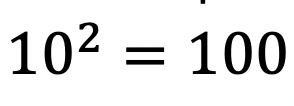). But what if it were to get more complicated?  That’s where logs come in!

## How to Solve Log Equations?

Logarithms follow a swooping pattern that allows us to write it in exponential form, let’s take a look at some Examples below:

But wait there’s more! Logs have a set of Rules that makes solving log equations a breeze!

We can use these rules to help us algebraically solve logarithmic equations, let’s look at an example that applies the Product Rule.

## Example:

Try the following practice questions on your own!

Practice Questions:

Solutions:

Still got questions?  No problem! Check out the video that goes over the same example outlined above.  And for more info. on logarithms check out this post that goes over a NYS Regent’s question here.  Subscribe below to get the latest FREE math videos, lessons, and practice questions from MathSux. Thanks for stopping by and happy calculating! 🙂

****Check out this Bonus Video on How to Change Log Bases****

## How to Graph Equation of a Line, y=mx+b: Algebra

Hi everyone, welcome back to Mathsux! This week we’ll be reviewing how to graph an equation of a line in y=mx+b form. And if you have not checked out the video below, please do! Happy calculating! 🙂

An equation of a line can be represented by the following formula:

y=mx+b

Y-Intercept: This is represented by b, the stand-alone number in y=mx+b. This represents where the line hits the y-axis.  This is always the first point you want to start with when graphing at coordinate point (0,b).

Slope: This is represented by m, the number next to x in y=mx+b. Slope tells us how much we go up or down the y-axis and left or right on the x- axis in fraction form:

Now let’s check out an Example!

Graph the following: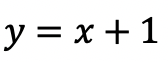-> First, let’s identify the slope and y-intercept of our line.

-> To start, let’s graph the first point on our graph, the y-intercept at point (0,1):

-> Now for the slope. We are going to go up one and over to the right one for each point, since our slope is 1/1.

-> Connect all of our coordinate points and label our graph.

Try the following practice questions on your own!

Practice Questions:

Want more Mathsux?  Don’t forget to check out our Youtube channel and more below! And if you have any questions, please don’t hesitate to comment below. Happy Calculating! 🙂

Need to brush up on slope? Click here to see how to find the rate of change.

## 4 Ways to Factor Trinomials: Algebra

Greeting math peeps and welcome to MathSux! In this post, we are going to go over 4 ways to Factor Trinomials and get the same answer, including, (1) Quadratic Formula (2) Product/Sum, (3) Completing the Square, and (4) Graphing on a Calculator.  If you’re looking for more don’t forget to check out the video and practice questions below.  Happy Calculating! 🙂

Also, if need a review on Factor by Grouping or Difference of Two Squares (DOTS) check out the hyperlinks here!

*If you haven’t done so, check out the video that goes over this exact problem, and don’t forget to subscribe!

We will take this step by step, showing 4 ways to factor trinomials, getting the same answer each and every time! Let’s get to it!

## 4 Ways to Factor Trinomials

____________________________________________________________________

## (2) Product/Sum: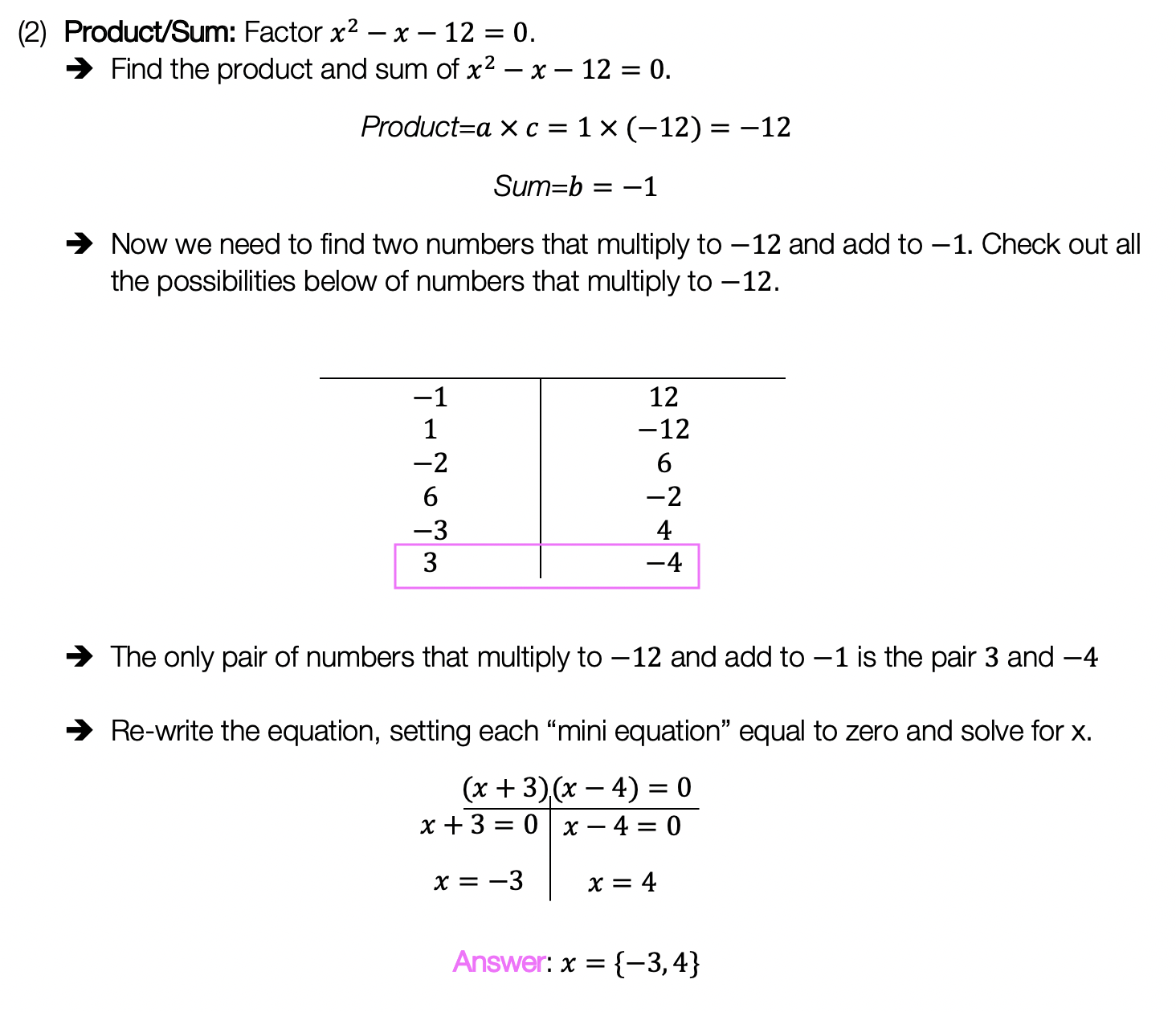____________________________________________________________________

## (3) Completing the Square: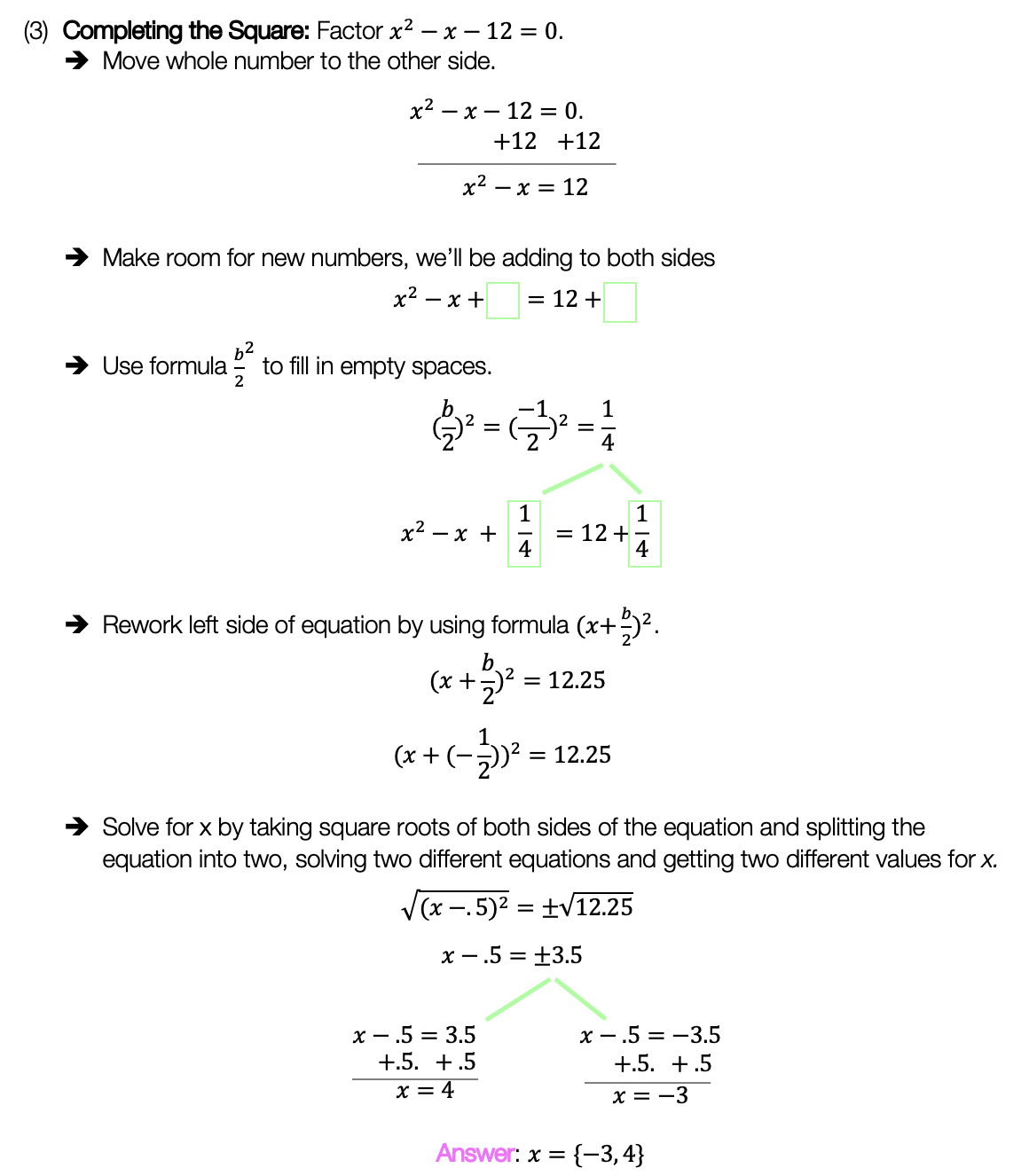____________________________________________________________________

## (4) Graph:

Choose the factoring method that works best for you and try the practice problems on your own below!

Practice Questions:

Solutions:

Want a review of all the different factoring methods out there?  Check out the ones left out here (DOTS and GCF) and happy calculating! 🙂

For even more ways to factor quadratic equations, check out How to factor by Grouping here! 🙂

Also, if you want more Mathsux?  Don’t forget to check out our Youtube channel and more below! If you have any questions, please don’t hesitate to comment below. Happy Calculating! 🙂

## Median of a Trapezoid Theorem: Geometry

Hi everyone and welcome to Math Sux! In this post, we are going to look at how to use and applythe median of a trapezoid theorem. Thankfully, it is not a scary formula, and one we can easily master with a dose of algebra. The only hard part remaining, is remembering this thing! Take a look below to see a step by step tutorial on how to use the median of a trapezoid theorem and check out the practice questions at the end of this post to truly master the topic. Happy calculating! 🙂

*If you haven’t done so, check out the video that goes over this exact problem, also please don’t forget to subscribe!

Step 1:  Let’s apply the Median of a Trapezoid Theorem to this question!  A little rusty?  No problem, check out the Theorem below.

Median of a Trapezoid Theorem: The median of a trapezoid is equal to the sum of both bases.Step 2: Now that we found the value of x , we can plug it back into the equation for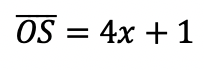median,  to find the value of median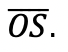Want more practice?  Your wish is my command! Check out the practice problems below:

Practice Questions:

1.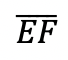is the median of trapezoid ABCDEF, find the value of the median, given the following:2.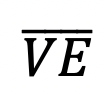is the median of trapezoid ACTIVE, find the value of the median, given the following:3.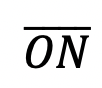is the median of  trapezoid DRAGON, find the value of the median, given the following:

4.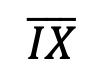is the median of trapezoid MATRIX, find the value of the median, given the following:

Solutions:

Need more of an explanation?  Check out the detailedand practice problems. Happy calculating! 🙂

## Completing the Square: Algebra

Want to learn the ins and out of completing the square?  Then you’ve come to the right place! Learn how to Complete the Square step by step in the video and article below, then try the practice problems at the end of this post to truly master the topic! If you’re looking for more on completing the square, check out this post here. Happy Calculating! 🙂 Check out the video below for an in-depth look at completing the square: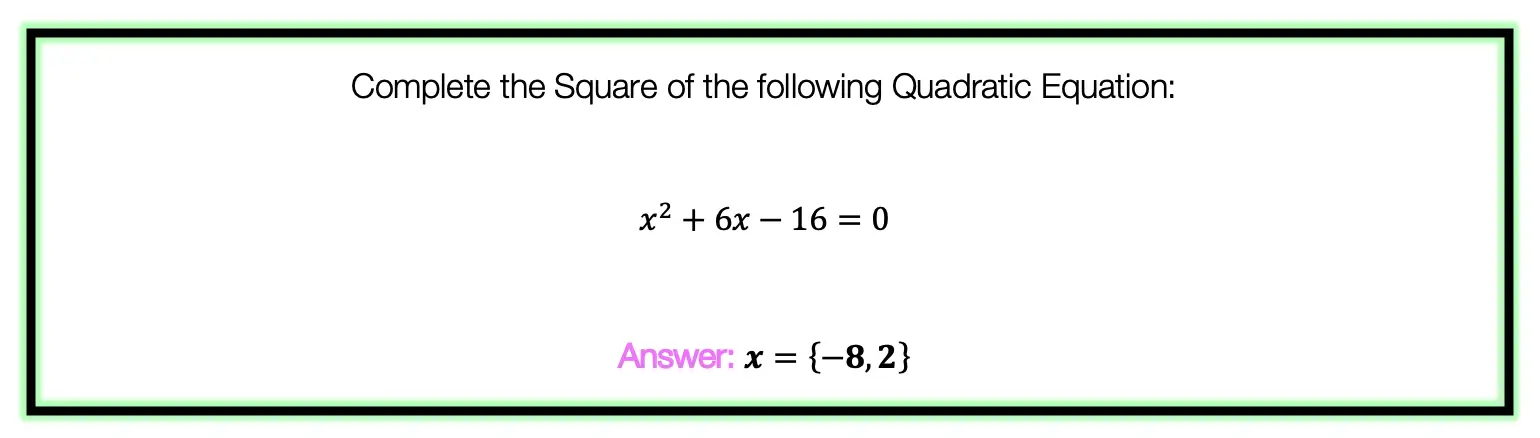To answer this question, there are several steps we must follow including: Step 1: Move the whole number, which in this case is 16, to the other side of the equation.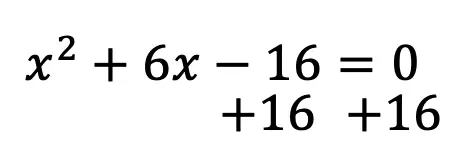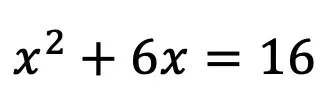Step 2: Make space for our new number on both sides of the equation.  This number is going to be found by using a particular formula shown below: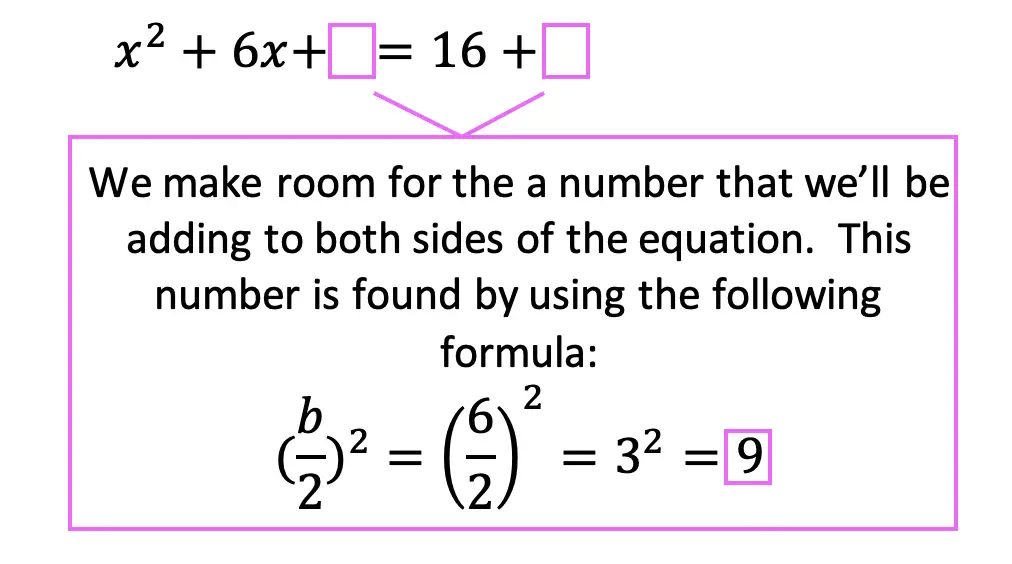Step 3: Add the number 9 to both sides of the equation, which we found using our formula.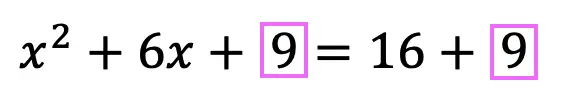Step 4: Combine like terms on the right side of the equation, adding 16+9 to get 25.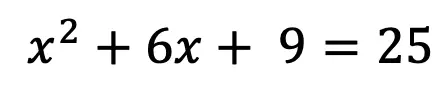Step 5: Now, we need to re-write the left side of the equation using the following formula.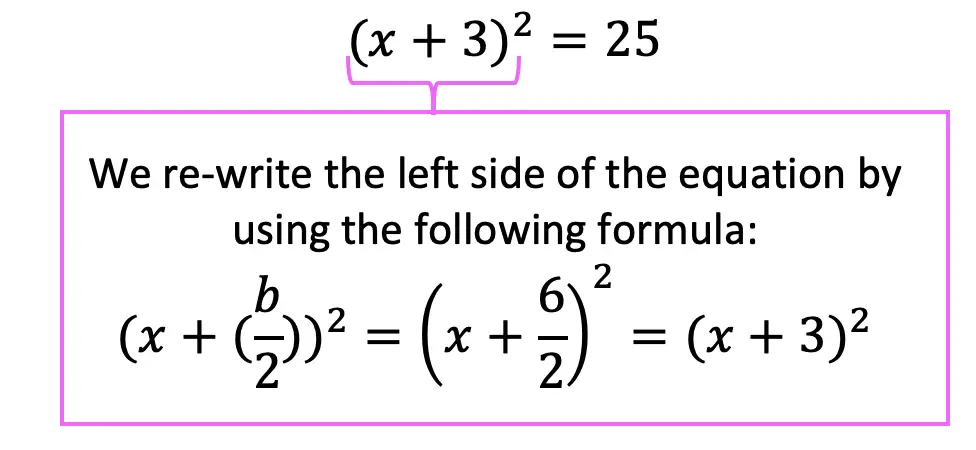Step 6: Finally, we solve for x by taking the positive and negative square root to get the following answer and solve for two different equations: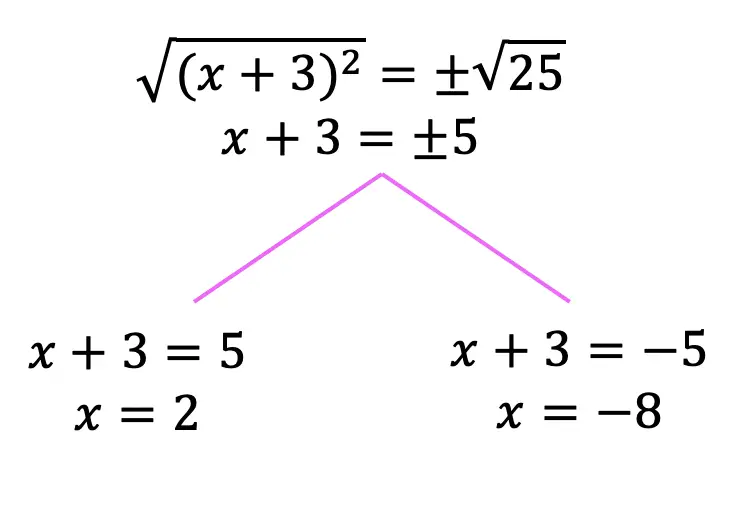Practice Questions: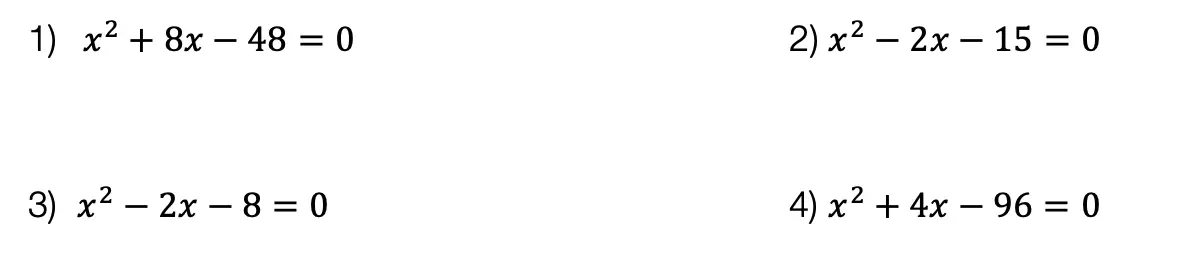Solutions: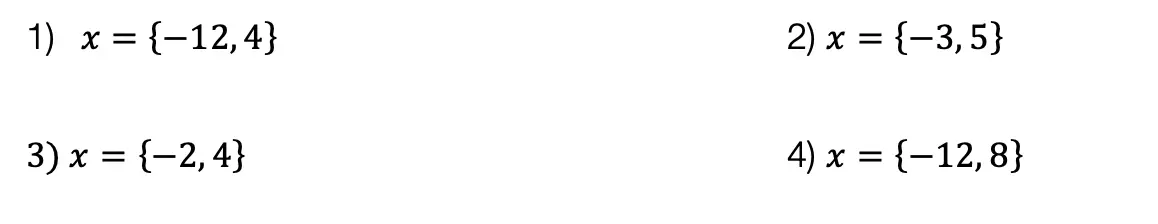Want more Mathsux?  Don’t forget to check out our Youtube channel and more below! And if you have any questions, please don’t hesitate to comment below. Happy Calculating! 🙂

Need more of an explanation?  Check out why we complete the square in the first place here ! 🙂